# Report on the experiment on the signal generated when a gentle flame is applied to the leaf of a tomato plant¶

In :
from IPython.core.display import Image
Image(filename='./flame/flame.jpg')

Out: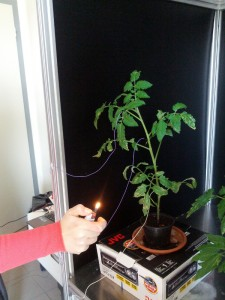In :
from numpy import *
from scipy import signal
from scipy.signal import filter_design as fd
from matplotlib import pyplot as plt
import scipy
import scipy.fftpack

In :
from nptdms import TdmsFile
tdms_file_EL1_ref = TdmsFile("./flame/data/experimentLS.tdms")
tdms_file_EL2_ref = TdmsFile("./flame/data/experimentPS.tdms")
'''
gruppi = tdms_file_EL2_ref.groups()
print gruppi
channels = tdms_file_EL2_ref.group_channels('Untitled')
print channels
'''
data1 = channel1.data
time1 = channel1.time_track()
channel2 = tdms_file_EL2_ref.object('Untitled', 'Voltage_1')
data2 = channel2.data
time2 = channel2.time_track()
# do stuff with data
#sample_per_sec = 1000
figsize(20,5)
figure(1)
plot(time1,data1)
figure(2)
plot(time2,data2)

Out:
[<matplotlib.lines.Line2D at 0x319fdf90>]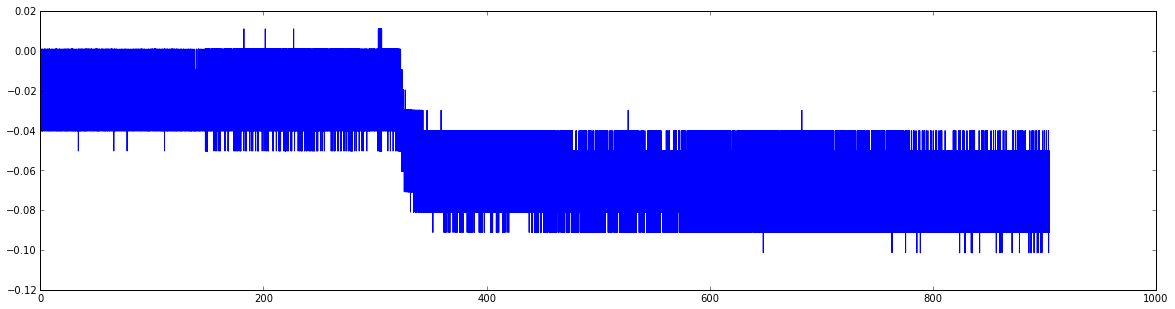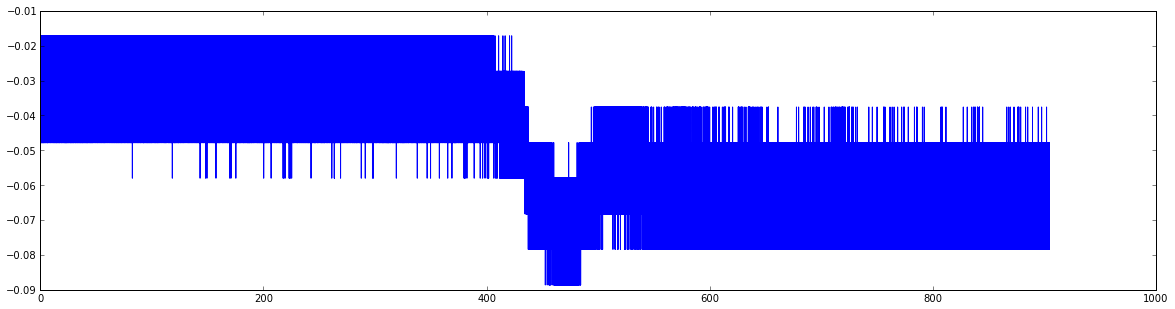In :
from scipy.signal import lfilter, firwin
#------------------------------------------------
# Create a FIR filter and apply it to signal.
#------------------------------------------------
# The Nyquist rate of the signal.
sample_rate = 1000.
nyq_rate = sample_rate / 2.

# The cutoff frequency of the filter: 6KHz = 6000.0
cutoff_hz = 0.2
#cutoff_hz = 0.003

# Length of the filter (number of coefficients, i.e. the filter order + 1)
numtaps = 29

# Use firwin to create a lowpass FIR filter
fir_coeff = firwin(numtaps, cutoff_hz/nyq_rate)

# Use lfilter to filter the signal with the FIR filter
filtered_data1 = lfilter(fir_coeff, 1.0, data1)
filtered_data2 = lfilter(fir_coeff, 1.0, data2)

In :
figsize(20,5)
figure(1)
plot(time1,filtered_data1)
figure(2)
plot(time2,filtered_data2)

Out:
[<matplotlib.lines.Line2D at 0x42725b90>]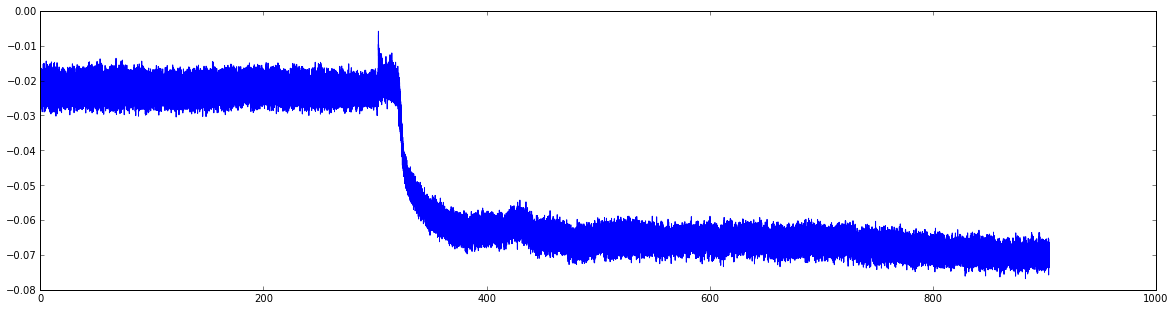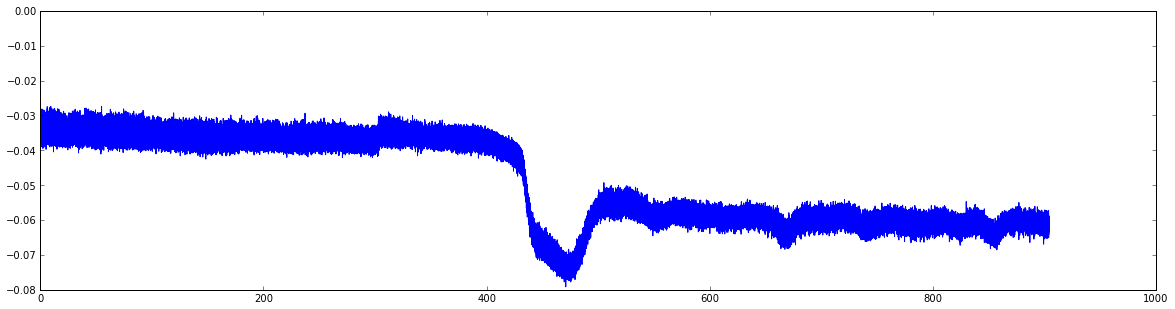The flame has been applied at time 300. Note the delay in the propagation of the signal which is about 2 minute (120 secs)